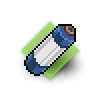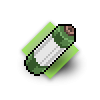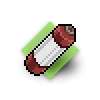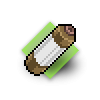# Furniture

## 57 products

1.\$2.90

2.\$2.90

3.\$2.90

4.\$2.90

5.\$2.90

6.\$2.90

7.\$2.90

8.\$2.90

9.\$2.90

10.\$2.90

11.\$2.90

12.\$2.90

13.\$2.90

14.\$2.90

15.\$2.90

16.\$2.90

17.\$2.90

18.\$2.90

19.\$2.90

20.\$2.90

21.\$2.90

22.\$2.90

23.\$2.90

24.\$2.90

25.\$2.90

26.\$2.90

27.\$2.90

28.\$2.90

29.\$2.90

30.\$2.90

31.\$2.90

32.\$2.90

33.\$2.90

34.\$2.90

35.\$2.90

36.\$2.90

37.\$2.90

38.\$2.90

39.\$2.90

40.\$2.90

41.\$2.90

42.\$2.90

43.\$2.90

44.\$2.90

45.\$2.90

46.\$2.90

47.\$2.90

48.\$2.90

49.\$2.90

50.\$2.90